## 5.23. among

Origin
Constraint

$\mathrm{𝚊𝚖𝚘𝚗𝚐}\left(\mathrm{𝙽𝚅𝙰𝚁},\mathrm{𝚅𝙰𝚁𝙸𝙰𝙱𝙻𝙴𝚂},\mathrm{𝚅𝙰𝙻𝚄𝙴𝚂}\right)$

Synonyms

$\mathrm{𝚋𝚎𝚝𝚠𝚎𝚎𝚗}$, $\mathrm{𝚌𝚘𝚞𝚗𝚝}$.

Arguments
 $\mathrm{𝙽𝚅𝙰𝚁}$ $\mathrm{𝚍𝚟𝚊𝚛}$ $\mathrm{𝚅𝙰𝚁𝙸𝙰𝙱𝙻𝙴𝚂}$ $\mathrm{𝚌𝚘𝚕𝚕𝚎𝚌𝚝𝚒𝚘𝚗}\left(\mathrm{𝚟𝚊𝚛}-\mathrm{𝚍𝚟𝚊𝚛}\right)$ $\mathrm{𝚅𝙰𝙻𝚄𝙴𝚂}$ $\mathrm{𝚌𝚘𝚕𝚕𝚎𝚌𝚝𝚒𝚘𝚗}\left(\mathrm{𝚟𝚊𝚕}-\mathrm{𝚒𝚗𝚝}\right)$
Restrictions
 $\mathrm{𝙽𝚅𝙰𝚁}\ge 0$ $\mathrm{𝙽𝚅𝙰𝚁}\le |\mathrm{𝚅𝙰𝚁𝙸𝙰𝙱𝙻𝙴𝚂}|$ $\mathrm{𝚛𝚎𝚚𝚞𝚒𝚛𝚎𝚍}$$\left(\mathrm{𝚅𝙰𝚁𝙸𝙰𝙱𝙻𝙴𝚂},\mathrm{𝚟𝚊𝚛}\right)$ $\mathrm{𝚛𝚎𝚚𝚞𝚒𝚛𝚎𝚍}$$\left(\mathrm{𝚅𝙰𝙻𝚄𝙴𝚂},\mathrm{𝚟𝚊𝚕}\right)$ $\mathrm{𝚍𝚒𝚜𝚝𝚒𝚗𝚌𝚝}$$\left(\mathrm{𝚅𝙰𝙻𝚄𝙴𝚂},\mathrm{𝚟𝚊𝚕}\right)$
Purpose

$\mathrm{𝙽𝚅𝙰𝚁}$ is the number of variables of the collection $\mathrm{𝚅𝙰𝚁𝙸𝙰𝙱𝙻𝙴𝚂}$ that take their value in $\mathrm{𝚅𝙰𝙻𝚄𝙴𝚂}$.

Example
$\left(3,〈4,5,5,4,1〉,〈1,5,8〉\right)$

The $\mathrm{𝚊𝚖𝚘𝚗𝚐}$ constraint holds since exactly 3 values of the collection of variables $〈4,5,5,4,1〉$ belong to the set of values $\left\{1,5,8\right\}$.

All solutions

Figure 5.23.1 gives all solutions to the following non ground instance of the $\mathrm{𝚊𝚖𝚘𝚗𝚐}$ constraint: ${V}_{1}\in \left[1,5\right]$, ${V}_{2}\in \left[3,9\right]$, ${V}_{3}\in \left[5,6\right]$, ${V}_{4}\in \left[2,3\right]$, $\mathrm{𝚊𝚖𝚘𝚗𝚐}$$\left(\mathbf{3},〈{V}_{1},{V}_{2},{V}_{3},{V}_{4}〉,〈\mathbf{2},\mathbf{4}〉\right)$.

##### Figure 5.23.1. All solutions corresponding to the non ground example of the $\mathrm{𝚊𝚖𝚘𝚗𝚐}$ constraint of the All solutions slot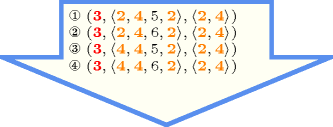Typical
 $\mathrm{𝙽𝚅𝙰𝚁}>0$ $\mathrm{𝙽𝚅𝙰𝚁}<|\mathrm{𝚅𝙰𝚁𝙸𝙰𝙱𝙻𝙴𝚂}|$ $|\mathrm{𝚅𝙰𝚁𝙸𝙰𝙱𝙻𝙴𝚂}|>1$ $|\mathrm{𝚅𝙰𝙻𝚄𝙴𝚂}|>1$ $|\mathrm{𝚅𝙰𝚁𝙸𝙰𝙱𝙻𝙴𝚂}|>|\mathrm{𝚅𝙰𝙻𝚄𝙴𝚂}|$
Symmetries
• Items of $\mathrm{𝚅𝙰𝚁𝙸𝙰𝙱𝙻𝙴𝚂}$ are permutable.

• Items of $\mathrm{𝚅𝙰𝙻𝚄𝙴𝚂}$ are permutable.

• An occurrence of a value of $\mathrm{𝚅𝙰𝚁𝙸𝙰𝙱𝙻𝙴𝚂}.\mathrm{𝚟𝚊𝚛}$ that belongs to $\mathrm{𝚅𝙰𝙻𝚄𝙴𝚂}.\mathrm{𝚟𝚊𝚕}$ (resp. does not belong to $\mathrm{𝚅𝙰𝙻𝚄𝙴𝚂}.\mathrm{𝚟𝚊𝚕}$) can be replaced by any other value in $\mathrm{𝚅𝙰𝙻𝚄𝙴𝚂}.\mathrm{𝚟𝚊𝚕}$ (resp. not in $\mathrm{𝚅𝙰𝙻𝚄𝙴𝚂}.\mathrm{𝚟𝚊𝚕}$).

Arg. properties
• Functional dependency: $\mathrm{𝙽𝚅𝙰𝚁}$ determined by $\mathrm{𝚅𝙰𝚁𝙸𝙰𝙱𝙻𝙴𝚂}$ and $\mathrm{𝚅𝙰𝙻𝚄𝙴𝚂}$.

• Contractible wrt. $\mathrm{𝚅𝙰𝚁𝙸𝙰𝙱𝙻𝙴𝚂}$ when $\mathrm{𝙽𝚅𝙰𝚁}=0$.

• Contractible wrt. $\mathrm{𝚅𝙰𝚁𝙸𝙰𝙱𝙻𝙴𝚂}$ when $\mathrm{𝙽𝚅𝙰𝚁}=|\mathrm{𝚅𝙰𝚁𝙸𝙰𝙱𝙻𝙴𝚂}|$.

• Aggregate: $\mathrm{𝙽𝚅𝙰𝚁}\left(+\right)$, $\mathrm{𝚅𝙰𝚁𝙸𝙰𝙱𝙻𝙴𝚂}\left(\mathrm{𝚞𝚗𝚒𝚘𝚗}\right)$, $\mathrm{𝚅𝙰𝙻𝚄𝙴𝚂}\left(\mathrm{𝚜𝚞𝚗𝚒𝚘𝚗}\right)$.

Remark

A similar constraint called $\mathrm{𝚋𝚎𝚝𝚠𝚎𝚎𝚗}$ was introduced in CHIP in 1990.

The $\mathrm{𝚌𝚘𝚖𝚖𝚘𝚗}$ constraint can be seen as a generalisation of the $\mathrm{𝚊𝚖𝚘𝚗𝚐}$ constraint where we allow the $\mathrm{𝚟𝚊𝚕}$ attributes of the $\mathrm{𝚅𝙰𝙻𝚄𝙴𝚂}$ collection to be domain variables.

A generalisation of this constraint when the values of $\mathrm{𝚅𝙰𝙻𝚄𝙴𝚂}$ are not initially fixed is called $\mathrm{𝚊𝚖𝚘𝚗𝚐}_\mathrm{𝚟𝚊𝚛}$.

When the variable $\mathrm{𝙽𝚅𝙰𝚁}$ (i.e., the first argument of the $\mathrm{𝚊𝚖𝚘𝚗𝚐}$ constraint) does not occur in any other constraints of the problem, it may be operationally more efficient to replace the $\mathrm{𝚊𝚖𝚘𝚗𝚐}$ constraint by an $\mathrm{𝚊𝚖𝚘𝚗𝚐}_\mathrm{𝚕𝚘𝚠}_\mathrm{𝚞𝚙}$ constraint where $\mathrm{𝙽𝚅𝙰𝚁}$ is replaced by the corresponding interval $\left[\underline{\mathrm{𝙽𝚅𝙰𝚁}},\overline{\mathrm{𝙽𝚅𝙰𝚁}}\right]$. This stands for two reasons:

It was shown in [ChabertDemassey12] that achieving bound-consistency for a conjunction of $\mathrm{𝚊𝚖𝚘𝚗𝚐}$ constraints where all sets of values are arbitrary intervals can be done in polynomial time.

Algorithm

A filtering algorithm achieving arc-consistency was given by Bessière et al. in [BessiereHebrardHnichKiziltanWalsh05ERCIM], [BessiereHebrardHnichKiziltanWalsh06ERCIM].

Systems

among in Choco, count in Gecode, among in JaCoP, among in MiniZinc.

generalisation: $\mathrm{𝚊𝚖𝚘𝚗𝚐}_\mathrm{𝚟𝚊𝚛}$ ($\mathrm{𝚌𝚘𝚗𝚜𝚝𝚊𝚗𝚝}$ replaced by $\mathrm{𝚟𝚊𝚛𝚒𝚊𝚋𝚕𝚎}$).

related: $\mathrm{𝚛𝚘𝚘𝚝𝚜}$ (can be used for expressing $\mathrm{𝚊𝚖𝚘𝚗𝚐}$), $\mathrm{𝚜𝚕𝚒𝚍𝚒𝚗𝚐}_\mathrm{𝚌𝚊𝚛𝚍}_\mathrm{𝚜𝚔𝚒𝚙}\mathtt{0}$ (counting constraint on maximal sequences).

shift of concept: $\mathrm{𝚊𝚖𝚘𝚗𝚐}_\mathrm{𝚜𝚎𝚚}$ ($\mathrm{𝚟𝚊𝚛𝚒𝚊𝚋𝚕𝚎}$ replaced by $\mathrm{𝚒𝚗𝚝𝚎𝚛𝚟𝚊𝚕}$ and constraint applied in a sliding way), $\mathrm{𝚌𝚘𝚖𝚖𝚘𝚗}$.

specialisation: $\mathrm{𝚊𝚖𝚘𝚗𝚐}_\mathrm{𝚍𝚒𝚏𝚏}_\mathtt{0}$ ($\mathrm{𝚟𝚊𝚛𝚒𝚊𝚋𝚕𝚎}\in \mathrm{𝚟𝚊𝚕𝚞𝚎𝚜}$ replaced by $\mathrm{𝚟𝚊𝚛𝚒𝚊𝚋𝚕𝚎}$ different from 0), $\mathrm{𝚊𝚖𝚘𝚗𝚐}_\mathrm{𝚒𝚗𝚝𝚎𝚛𝚟𝚊𝚕}$ ($\mathrm{𝚟𝚊𝚛𝚒𝚊𝚋𝚕𝚎}\in \mathrm{𝚟𝚊𝚕𝚞𝚎𝚜}$ replaced by $\mathrm{𝚟𝚊𝚛𝚒𝚊𝚋𝚕𝚎}\in \mathrm{𝚒𝚗𝚝𝚎𝚛𝚟𝚊𝚕}$), $\mathrm{𝚊𝚖𝚘𝚗𝚐}_\mathrm{𝚕𝚘𝚠}_\mathrm{𝚞𝚙}$ ($\mathrm{𝚟𝚊𝚛𝚒𝚊𝚋𝚕𝚎}$ replaced by $\mathrm{𝚒𝚗𝚝𝚎𝚛𝚟𝚊𝚕}$), $\mathrm{𝚊𝚖𝚘𝚗𝚐}_\mathrm{𝚖𝚘𝚍𝚞𝚕𝚘}$ (list of $\mathrm{𝚟𝚊𝚕𝚞𝚎𝚜}$ replaced by list of $\mathrm{𝚟𝚊𝚕𝚞𝚎𝚜}$ $𝚟$ such that $𝚟\mathrm{mod}\mathrm{𝚀𝚄𝙾𝚃𝙸𝙴𝙽𝚃}$ = $\mathrm{𝚁𝙴𝙼𝙰𝙸𝙽𝙳𝙴𝚁}$), $\mathrm{𝚎𝚡𝚊𝚌𝚝𝚕𝚢}$ ($\mathrm{𝚟𝚊𝚛𝚒𝚊𝚋𝚕𝚎}$ replaced by $\mathrm{𝚌𝚘𝚗𝚜𝚝𝚊𝚗𝚝}$ and $\mathrm{𝚟𝚊𝚕𝚞𝚎𝚜}$ replaced by one single $\mathrm{𝚟𝚊𝚕𝚞𝚎}$).

system of constraints: $\mathrm{𝚐𝚕𝚘𝚋𝚊𝚕}_\mathrm{𝚌𝚊𝚛𝚍𝚒𝚗𝚊𝚕𝚒𝚝𝚢}$ (count the number of occurrences of different values).

Keywords
Arc input(s)

$\mathrm{𝚅𝙰𝚁𝙸𝙰𝙱𝙻𝙴𝚂}$

Arc generator
$\mathrm{𝑆𝐸𝐿𝐹}$$↦\mathrm{𝚌𝚘𝚕𝚕𝚎𝚌𝚝𝚒𝚘𝚗}\left(\mathrm{𝚟𝚊𝚛𝚒𝚊𝚋𝚕𝚎𝚜}\right)$

Arc arity
Arc constraint(s)
$\mathrm{𝚒𝚗}$$\left(\mathrm{𝚟𝚊𝚛𝚒𝚊𝚋𝚕𝚎𝚜}.\mathrm{𝚟𝚊𝚛},\mathrm{𝚅𝙰𝙻𝚄𝙴𝚂}\right)$
Graph property(ies)
$\mathrm{𝐍𝐀𝐑𝐂}$$=\mathrm{𝙽𝚅𝙰𝚁}$

Graph model

The arc constraint corresponds to the unary constraint $\mathrm{𝚒𝚗}\left(\mathrm{𝚟𝚊𝚛𝚒𝚊𝚋𝚕𝚎𝚜}.\mathrm{𝚟𝚊𝚛},\mathrm{𝚅𝙰𝙻𝚄𝙴𝚂}\right)$ defined in this catalogue. Since this is a unary constraint we employ the $\mathrm{𝑆𝐸𝐿𝐹}$ arc generator in order to produce an initial graph with a single loop on each vertex.

Parts (A) and (B) of Figure 5.23.2 respectively show the initial and final graph associated with the Example slot. Since we use the $\mathrm{𝐍𝐀𝐑𝐂}$ graph property, the loops of the final graph are stressed in bold.

##### Figure 5.23.2. Initial and final graph of the $\mathrm{𝚊𝚖𝚘𝚗𝚐}$ constraint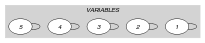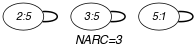(a) (b)
Automaton

Figure 5.23.3 depicts a first automaton that only accepts all the solutions to the $\mathrm{𝚊𝚖𝚘𝚗𝚐}$ constraint. This automaton uses a counter in order to record the number of satisfied constraints of the form ${\mathrm{𝚅𝙰𝚁}}_{i}\in \mathrm{𝚅𝙰𝙻𝚄𝙴𝚂}$ already encountered. To each variable ${\mathrm{𝚅𝙰𝚁}}_{i}$ of the collection $\mathrm{𝚅𝙰𝚁𝙸𝙰𝙱𝙻𝙴𝚂}$ corresponds a 0-1 signature variable ${S}_{i}$. The following signature constraint links ${\mathrm{𝚅𝙰𝚁}}_{i}$ and ${S}_{i}$: ${\mathrm{𝚅𝙰𝚁}}_{i}\in \mathrm{𝚅𝙰𝙻𝚄𝙴𝚂}⇔{S}_{i}$. The automaton counts the number of variables of the $\mathrm{𝚅𝙰𝚁𝙸𝙰𝙱𝙻𝙴𝚂}$ collection that take their value in $\mathrm{𝚅𝙰𝙻𝚄𝙴𝚂}$ and finally assigns this number to $\mathrm{𝙽𝚅𝙰𝚁}$.

##### Figure 5.23.3. Automaton (with one counter) of the $\mathrm{𝚊𝚖𝚘𝚗𝚐}$ constraint and its glue matrix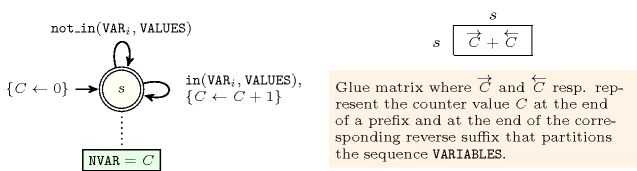##### Figure 5.23.4. Hypergraph of the reformulation corresponding to the automaton (with one counter) of the $\mathrm{𝚊𝚖𝚘𝚗𝚐}$ constraint: since all states variables ${Q}_{0},{Q}_{1},\cdots ,{Q}_{n}$ are fixed to the unique state $s$ of the automaton, the transitions constraints share only the counter variable $C$ and the constraint network is Berge-acyclic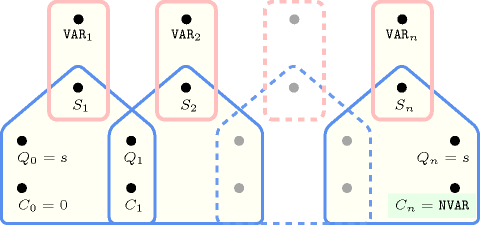We now describe a second counter free automaton that also only accepts all the solutions to $\mathrm{𝚊𝚖𝚘𝚗𝚐}$ constraint. Without loss of generality, assume that the collection of variables $\mathrm{𝚅𝙰𝚁𝙸𝙰𝙱𝙻𝙴𝚂}$ contains at least one variable (i.e., $|\mathrm{𝚅𝙰𝚁𝙸𝙰𝙱𝙻𝙴𝚂}|\ge 1$). Let $n$ and $𝒟$ respectively denote the number of variables of the collection $\mathrm{𝚅𝙰𝚁𝙸𝙰𝙱𝙻𝙴𝚂}$, and the union of the domains of the variables of $\mathrm{𝚅𝙰𝚁𝙸𝙰𝙱𝙻𝙴𝚂}$. Clearly, the maximum number of variables of $\mathrm{𝚅𝙰𝚁𝙸𝙰𝙱𝙻𝙴𝚂}$ that are assigned a value in $\mathrm{𝚅𝙰𝙻𝚄𝙴𝚂}$ cannot exceed the quantity $m=min\left(n,\overline{\mathrm{𝙽𝚅𝙰𝚁}}\right)$. The $m+2$ states of the automaton that only accepts all the solutions to the $\mathrm{𝚊𝚖𝚘𝚗𝚐}$ constraint can be defined in the following way:

• We have an initial state labelled by ${s}_{0}$.

• We have $m$ intermediate states labelled by ${s}_{i}$ $\left(1\le i\le m\right)$. The intermediate states are indexed by the number of already encountered satisfied constraints of the form ${\mathrm{𝚅𝙰𝚁}}_{k}\in \mathrm{𝚅𝙰𝙻𝚄𝙴𝚂}$ from the initial state ${s}_{0}$ to the state ${s}_{i}$.

• We have an accepting state labelled by ${s}_{F}$.

Three classes of transitions are respectively defined in the following way:

1. There is a transition, labeled by $j$, $\left(j\in 𝒟\setminus \mathrm{𝚅𝙰𝙻𝚄𝙴𝚂}\right)$, from every state ${s}_{i}$, $\left(i\in \left[0,m\right]\right)$, to itself.

2. There is a transition, labeled by $j$, $\left(j\in \mathrm{𝚅𝙰𝙻𝚄𝙴𝚂}\right)$, from every state ${s}_{i}$, $\left(i\in \left[0,m-1\right]\right)$, to the state ${s}_{i+1}$.

3. There is a transition, labelled by $i$, from every state ${s}_{i}$, $\left(i\in \left[0,m\right]\right)$, to the accepting state ${s}_{F}$.

This leads to an automaton that has $m·|𝒟|+|𝒟\setminus \mathrm{𝚅𝙰𝙻𝚄𝙴𝚂}|+m+1$ transitions. Since the maximum value of $m$ is equal to $n$, in the worst case we have $n·|𝒟|+|𝒟\setminus \mathrm{𝚅𝙰𝙻𝚄𝙴𝚂}|+n+1$ transitions.

Figure 5.23.5 depicts a counter free non deterministic automaton associated with the $\mathrm{𝚊𝚖𝚘𝚗𝚐}$ constraint under the hypothesis that (1) all variables of $\mathrm{𝚅𝙰𝚁𝙸𝙰𝙱𝙻𝙴𝚂}$ are assigned a value in $\left\{0,1,2,3\right\}$, (2) $|\mathrm{𝚅𝙰𝚁𝙸𝙰𝙱𝙻𝙴𝚂}|$ is equal to 3, (3) $\mathrm{𝚅𝙰𝙻𝚄𝙴𝚂}$ corresponds to odd values. The sequence ${\mathrm{𝚅𝙰𝚁}}_{1},{\mathrm{𝚅𝙰𝚁}}_{2},\cdots ,{\mathrm{𝚅𝙰𝚁}}_{|\mathrm{𝚅𝙰𝚁𝙸𝙰𝙱𝙻𝙴𝚂}|},\mathrm{𝙽𝚅𝙰𝚁}$ is passed to this automaton. A state ${s}_{i}$ $\left(1\le i\le 3\right)$ represents the fact that $i$ odd values were already encountered, while ${s}_{F}$ represents the accepting state. A transition from ${s}_{i}$ $\left(1\le i\le 3\right)$ to ${s}_{F}$ is labelled by $i$ and represents the fact that we can only go in the accepting state from a state that is compatible with the total number of odd values enforced by $\mathrm{𝙽𝚅𝙰𝚁}$. Note that non determinism only occurs if there is a non-empty intersection between the set of potential values that can be assigned to the variables of $\mathrm{𝚅𝙰𝚁𝙸𝙰𝙱𝙻𝙴𝚂}$ and the potential value of the $\mathrm{𝙽𝚅𝙰𝚁}$. While the counter free non deterministic automaton depicted by Figure 5.23.5 has 5 states and 18 transitions, its minimum-state deterministic counterpart shown in Figure 5.23.6 has 7 states and 23 transitions.

##### Figure 5.23.5. Counter free non deterministic automaton of the $\mathrm{𝚊𝚖𝚘𝚗𝚐}$$\left(\mathrm{𝙽𝚅𝙰𝚁},〈{\mathrm{𝚅𝙰𝚁}}_{1},{\mathrm{𝚅𝙰𝚁}}_{2},{\mathrm{𝚅𝙰𝚁}}_{3}〉,〈1,3〉\right)$ constraint assuming ${\mathrm{𝚅𝙰𝚁}}_{i}\in \left[0,3\right]$ $\left(1\le i\le 3\right)$, with initial state ${s}_{0}$ and accepting state ${s}_{F}$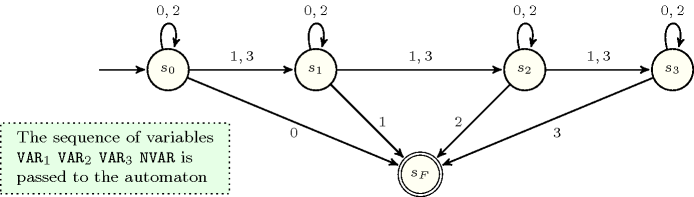##### Figure 5.23.6. Counter free minimum-state deterministic automaton of the $\mathrm{𝚊𝚖𝚘𝚗𝚐}$$\left(\mathrm{𝙽𝚅𝙰𝚁},〈{\mathrm{𝚅𝙰𝚁}}_{1},{\mathrm{𝚅𝙰𝚁}}_{2},{\mathrm{𝚅𝙰𝚁}}_{3}〉,〈1,3〉\right)$ constraint assuming ${\mathrm{𝚅𝙰𝚁}}_{i}\in \left[0,3\right]$ $\left(1\le i\le 3\right)$, with initial state ${s}_{0}$ and accepting states ${s}_{1}$, ${s}_{3}$, ${s}_{6}$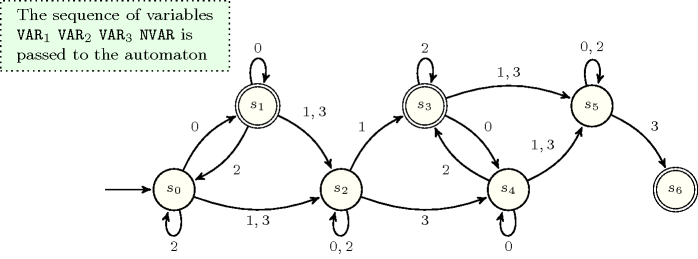We make the following final observation. Since the Symmetries slot of the $\mathrm{𝚊𝚖𝚘𝚗𝚐}$ constraint indicates that the variables of $\mathrm{𝚅𝙰𝚁𝙸𝙰𝙱𝙻𝙴𝚂}$ are permutable, and since all incoming transitions to any state of the automaton depicted by Figure 5.23.5 are labelled with distinct values, we can mechanically construct from this automaton a counter free deterministic automaton that takes as input the sequence $\mathrm{𝙽𝚅𝙰𝚁}$, ${\mathrm{𝚅𝙰𝚁}}_{3}$, ${\mathrm{𝚅𝙰𝚁}}_{2}$, ${\mathrm{𝚅𝙰𝚁}}_{1}$ rather than the sequence ${\mathrm{𝚅𝙰𝚁}}_{1}$, ${\mathrm{𝚅𝙰𝚁}}_{2}$, ${\mathrm{𝚅𝙰𝚁}}_{3}$, $\mathrm{𝙽𝚅𝙰𝚁}$. This is achieved by respectively making ${s}_{F}$ and ${s}_{0}$ the initial and the accepting state, and by reversing each transition.